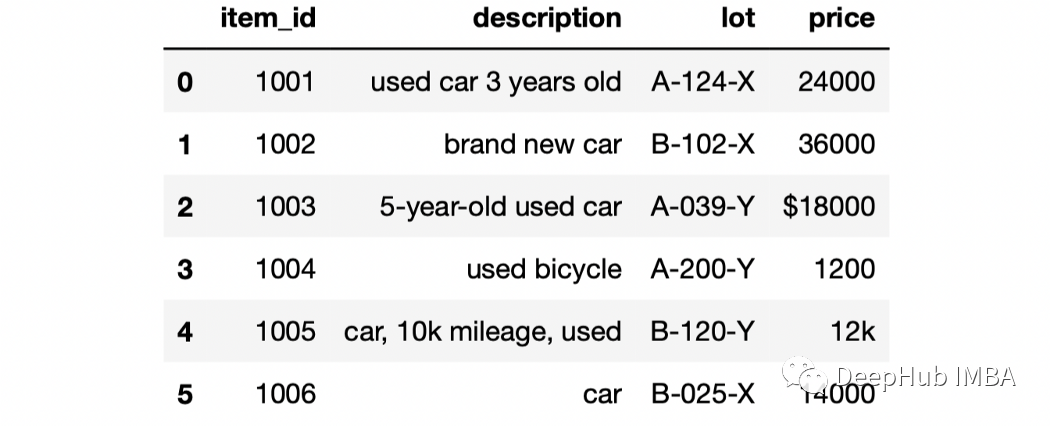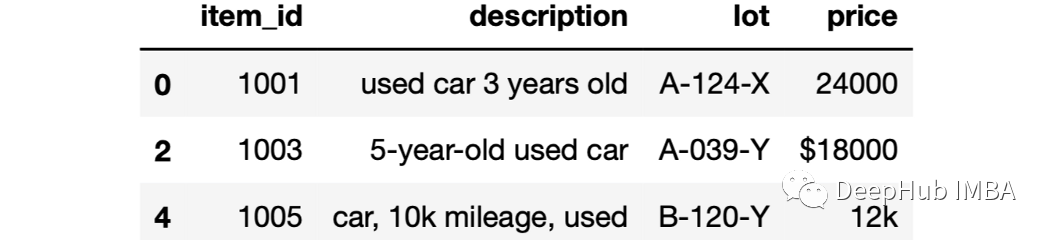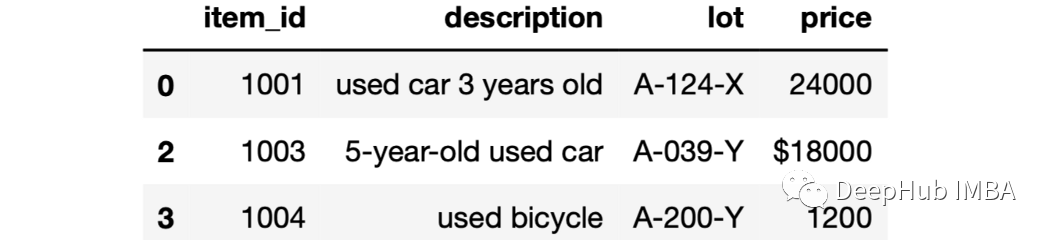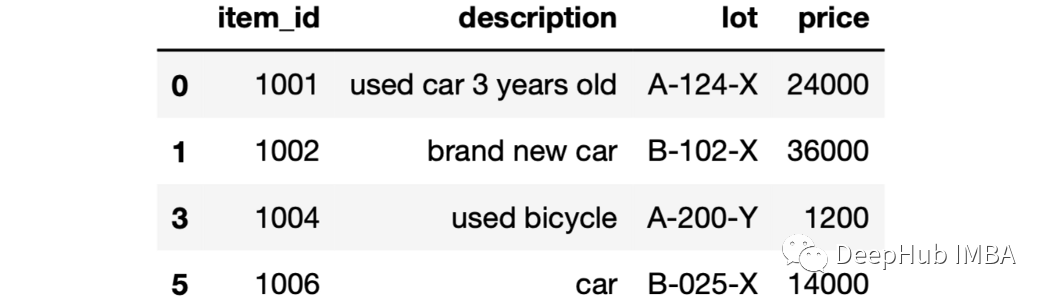0

# 5个例子学会Pandas中的字符串过滤

Pandas 库有许多可以轻松简单地处理文本数据函数和方法。在本文中，我介绍将学习 5 种可用于过滤文本数据（即字符串）的不同方法：

• 是否包含一系列字符
• 求字符串的长度
• 判断以特定的字符序列开始或结束
• 判断字符为数字或字母数字
• 查找特定字符序列的出现次数

`````` import pandas as pd
df
```````````` df[df["description"].str.contains("used car")]
```````````` df[df["description"].str.contains("used") &
df["description"].str.contains("car")]
```````````` df[df["description"].apply(lambda x: len(x) > 15)]
``````

`````` df[df["description"].str.len() > 15]
```````````` df[df["lot"].str.startswith("A")]
```````````` df[df["lot"].str.startswith("A-0")]
``````Python 的内置的字符串函数都可以应用到Pandas DataFrames 中。例如，在价格列中，有一些非数字字符，如 \$ 和 k。我们可以使用 isnumeric 函数过滤掉。

`````` df[df["price"].apply(lambda x: x.isnumeric()==True)]
``````count 方法可以计算单个字符或字符序列的出现次数。例如，查找一个单词或字符出现的次数。

`````` df["description"].str.count("used")

# 结果
0    1
1    0
2    1
3    1
4    1
5    0
Name: description, dtype: int64
``````

`````` df[df["description"].str.count("used") < 1]
``````

### “5个例子学会Pandas中的字符串过滤”的评论:

##### 关于作者##### Deephub